﻿ 高等数学——不定积分

## 高等数学——不定积分

' : 代表对F(x)求导（F'(x)）
d: 代表对F(x)求导（dF(x)），dx代表积分变量是x
f(x)dx: f(x)是导函数，dx表示求导变量是x

[G(x) - F(x)]' = G'(x) - F'(x) = f(x) - f(x) = 0
G(x) - F(x) = C
G(x) = F(x) + C

(1) [∫ƒ(x)dx]' = f(x) 或 d∫ƒ(x)dx = ƒ(x)dx
(2) ∫ƒ'(x)dx = ƒ(x) + C 或 ∫dƒ(x) = ƒ(x) + C

设F(x)是f(x)的一个原函数，则
[∫ƒ(x)dx]' = [F(x) + C]' = F'(x) = f(x)

∫[ƒ(x) + g(x)]dx = ∫ƒ(x)dx + ∫g(x)dx

将上式右端对x求导，得
[∫ƒ(x)dx + ∫g(x)dx]' = [∫ƒ(x)dx]' + [∫g(x)dx]' = ƒ(x) + g(x)

∫kƒ(x)dx = k∫ƒ(x)dx (k为非零常数)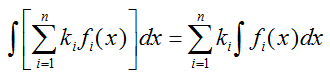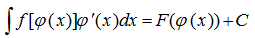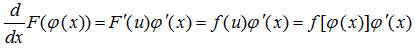(1) x=ψ(t)单调可微，且ψ'(t)≠0
(2) g[ψ(t)]ψ'(t)具有原函数F(t)。

∫g(x)dx=∫g[ψ(t)]ψ'(t)dt=F(t)+C=F(ψ-1(x))+C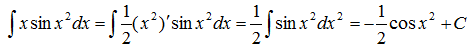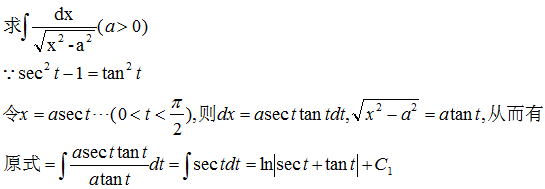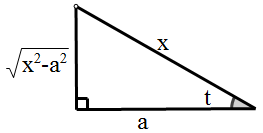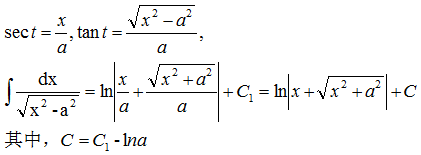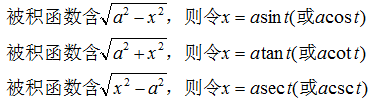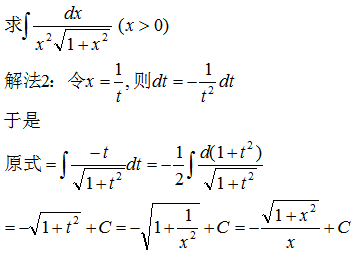(1) 选择 u，使它的导数 u' 比 u “简单”；<
(2) 选取 dv (或v') ，使 v 容易求出；
(3) 积分 ∫vdu 较易